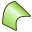# NU Card

This card defines surface triangles representing a NURBS surface.

On the Construct tab, in the Surfaces group, click theNURBS (NU) icon.

## Parameters:

Degree (p) of Bezier curve in U direction
The degree of the Bézier curve in the U 方向.
Degree (q) of Bezier curve in V direction
The degree of the Bézier curve in the V 方向.
Both p and q must be in the range from 1 to 4 where 1 is linear, 2 quadratic, and so forth. The control points are entered in the table, more or less representing their physical relation. There are $\text{p+1}$ rows and $\text{q+1}$ columns.

It is possible to create a triangular NURBS surface. In this case all control points on one side must be identical (use the same point). The weights of the control points are specified at the DP card. Note that for higher order Bézier curves, the surface does not pass through the control points except those on the corners.

## Examples of NU card usage:

The “saddle” point shape is generated with the NU card using 4 and 6 control points respectively.

The linear-quadratic shape is also generated with the NU card using 4 and 6 control points respectively.

NURBS may also be used to generate flat surfaces with curved edges. The section of a circular plate with a square hole is generated using the NU card.